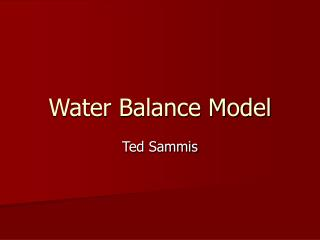DownloadDownload PresentationWater Balance Model

# Water Balance Model

Download Presentation## Water Balance Model

- - - - - - - - - - - - - - - - - - - - - - - - - - - E N D - - - - - - - - - - - - - - - - - - - - - - - - - - -
##### Presentation Transcript

1. Water Balance Model Ted Sammis

2. Water Balance • I+R-D-Et+- del SM=0 • I= irrigation inches 1-4 inches • R= rainfall inches = 0-1 inches • Et= evapotranspiration 0.4 inches/day • Del SM= change in soil moisture inches Time step 1 day

3. I+R-D-Et+- del SM=0 • Del SM= SMi- SMi-1 • Substitute into equation • SMi= I+R-D-Et+SMi-1 • Et= kc Eto • SMi= I+R-D- kc Eto +SMi-1

4. Example • I=0 R=0 D=0 kc=1 Eto= 0.4 inches FC= 0.3 inches/inch of soil Rd= 36 inches • Start at field capacity • SMi-1= Pv Rd= 0.3*36=10.8 inches • SMi= I+R-D- kc Eto +SMi-1 • SMi= 0+0-0-1*0.4+10.8= 10.4 inches

5. Exampleday two • I=0.0 R=0.1 D=0 kc=1 Eto= 0.4 inches SMi-1=10.4 inches • SMi= I+R-D- kc Eto +SMi-1 • SMi= 0.0+0.1-0-1*0.4+10.4= 10.1 inches

6. Exampleday three • I=1.5 R=0.0 D=0 kc=1 Eto= 0.4 inches • SMi-1=10.1 inches • SMi= I+R-D- kc Eto +SMi-1 • SMi= 1.5+0-0-1*0.4+10.1= 11.2 inches • SMi is greater than Field Capacity=10.8 • Reset SMi to 10.8 and D= 11.2-10.8=0.4 • Check calculation • SMi= 1.5+0-0.4-1*0.4+10.1= 10.8 inches

7. Water Balance • Water balance gets reset every time irrigation is enough to fill the root zone to field capacity. • Error in Et accumulates only between irrigations then resets.